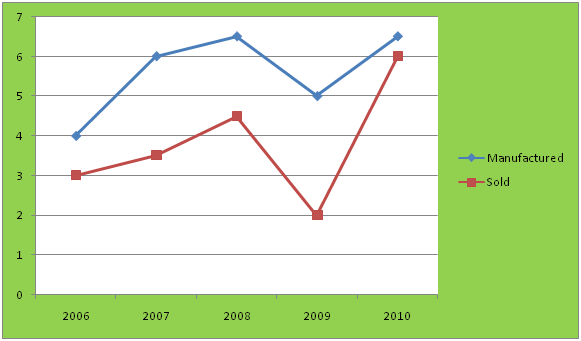# Techniques to solve Data Interpretation problems

Today I'm going to share problems related to X-Y charts in Data Interpretation. You all know that data interpretation is very scoring section if you can do calculations fast. Always remember that while doing the DI problems, just do the required calculations and if calculations are very lengthy, then try to find approximate value.

Following is a problem of X-Y chart. I guess you all know how the data is presented in X-Y charts. Lets try this problem. I will tell you the good way to solve the question.

Problem1: Study the following graph and try to answer the questions:

Graph: Units of products Manufactured and Sold by a company over the years (Units in lac).X axis to Years     Y axis to Units of products.

1. Find the average number of units sold over the years?

2. Find the ratio of difference between the number of units manufactured and sold in year 2008 to the difference between the number of units manufactured and sold in year 2009.

3. Find the ratio of number of units of manufactured in year 2007 to number of units manufactured in year 2009.

4. What is the approximate per cent increase in number of units sold in year 2010 from previous year?

5. What is the difference between the number of units manufactured and number of units sold over the years?

Solution: Study the graph first:
• One line is presenting units of manufactured products and other units of sold products.
• Manufacture - Sold = Products left
You can also make a table from chart if reading a chart seems difficult to you AS FOLLOWS:

 YEAR Units manufactured(M) Units Sold(S) Difference(M-S) 2006 4 3 1 2007 6 3.5 2.5 2008 6.5 4.5 2 2009 5 2 3 2010 6.5 6 0.5

I just rewrite the values from chart. If you can easily read the chart that there is no need to make the table.
Lets start solving the questions.

1. Average number of units sold over the years = (3+ 3.5+ 4.5+ 2+ 6)/5 = (19)/5 = 3.8 lac

2. To find: Ratio of difference between the number of units manufactured and sold in year 2008 to the difference between the number of units manufactured and sold in year 2009.

See the above table: Difference in 2008 = 2
Difference in 2009 = 3

Therefore, Ratio will be = 2:3

3. To find: Ratio of number of units of manufactured in year 2007 to number of units manufactured in year 2009.

Number of units manufactured in 2007 = 6
Number of units manufactured in 2009 = 5

Therefore, Ratio = 6:5

4.To find: Approximate per cent increase in number of units sold in year 2010 from previous year

Number of units sold in 2010 = 6
Number of units sold in 2009 = 2
per cent increase = (6-2)/2 times 100 = 200%

5. To find: Difference between the number of units manufactured and number of units sold over the years.

Number of units manufactured = 4+ 6+ 6.5+ 5+ 6.5 = 28
Number of sold = 3+ 3.5+ 4.5+ 2+ 6 = 19

Difference = 28- 19 = 9 lac.

I hope this article would help you to understand X-Y chart problems of Data Interpretation.

Also check the following article:
Data Interpretation: Tables
Data Interpretation: Bar Charts
Data Interpretation: Problems for practice

#### What's trending in BankExamsToday

Smart Prep Kit for Banking Exams by Ramandeep Singh - Download here

##### Join 40,000+ readers and get free notes in your email#### 1 comment:

1.thanks for informing us ..can u provide more such shortcuts to solve DI problems..for me the calculation takes lots of time .It would be very helpful if u can provide more

I will try to respond asap